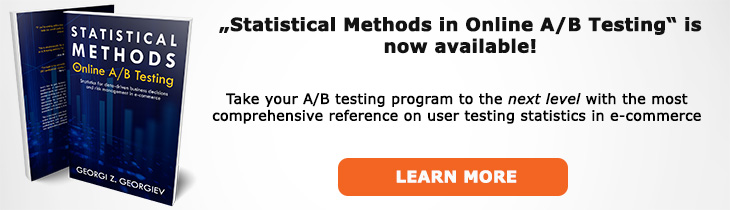# What does "Likelihood" mean?

Definition of Likelihood in the context of A/B testing (online controlled experiments).

## What is Likelihood?

Aliases: likelihood function

In frequentist inference the likelihood is a quantity proportional to the probability that, from a population having a particular value of θ, a sample having the observed value x0, should be obtained. Likelihood, being the outcome of a likelihood function thus defined, describes the plausibility, under a certain statistical model (the null hypothesis in hypothesis testing), of a certain parameter value after observing a particular outcome. Formally: L(θ; x0) ∝ ƒ(x0; θ), ∀θ∈Θ .

Likelihood is central to parametric statistical inference. The likelihood is a basis for the likelihood ratio test: a uniformly most powerful test for comparing two point hypotheses. It is also the basis for the maximum likelihood estimate.

In practice one often calculates the natural logarithm of the likelihood function (log-likelihood) as being more convenient (easier to differentiate). The fact that a logarithm is strictly increasing is useful when calculating maximum likelihood: log-likelihood reaches the maximum at the same point as the likelihood.

In Bayesian inference likelihood has a different meaning as the conditional probability P(E|H): the probability of evidence E given H, conditioning on an non-observable entity. The likelihood, being a conditional density can be multiplied by the prior probability density of the parameter and then normalized, producing the posterior probability.

## Related A/B Testing terms

Maximum Likelihood Estimate

Like this glossary entry? For an in-depth and comprehensive reading on A/B testing stats, check out the book "Statistical Methods in Online A/B Testing" by the author of this glossary, Georgi Georgiev.## Glossary Index by Letter

Select a letter to see all A/B testing terms starting with that letter or visit the Glossary homepage to see all.